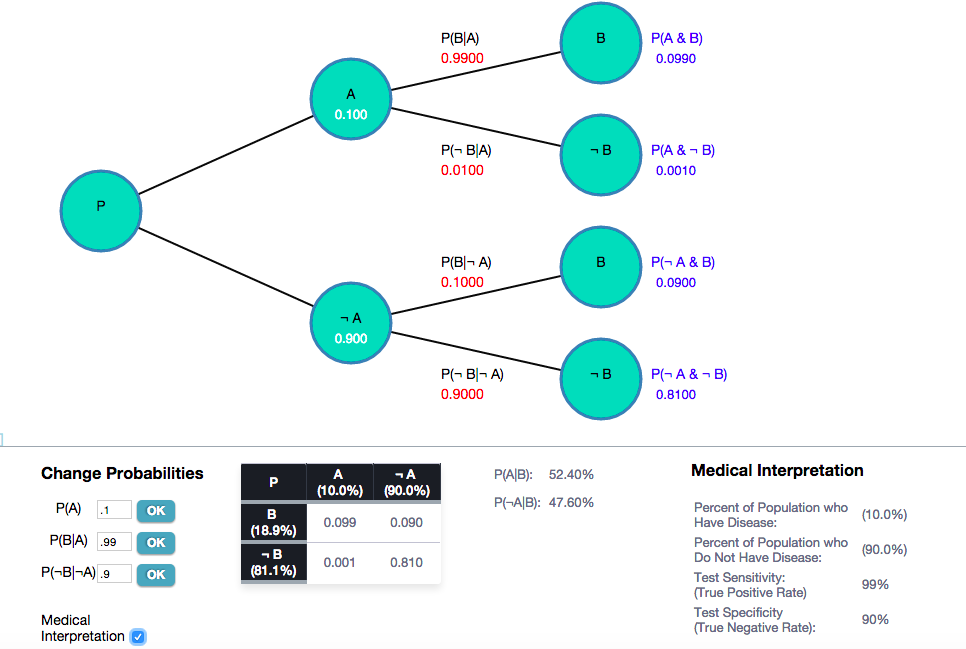# Probability Tree Diagrams Using D3 and Javascript

### Probability Tree Diagram

This post will discuss an Interactive Conditional Probability Tree Diagram that I created and how and why to do it.

### Conditional Probability and Probability Trees

I include some basic probability theory as part of a Problem Solving Course that I teach to law students.  Probability can be a useful skill for law students to learn given that attorneys are often called upon to make decisions in environments of uncertainty.

In teaching my students about Conditional Probability, it is often helpful to create a Conditional Probability Tree diagram like the one pictured above. Probability Tree diagrams can help the students visualize the branching structure of conditional probability.

### Probability Tree Diagrams Using D3 and Javascript

To create the interactive conditional probability tree diagram, I used the excellent D3 Data Framework and Javascript.The diagram automatically computes the relevant conditional probabilities given the input data.  It also allows you to change the input probabilities and recompute.